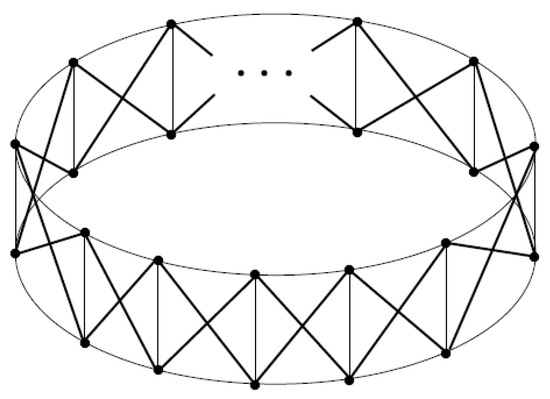Next Article in Journal
A New Extension of the τ-Gauss Hypergeometric Function and Its Associated Properties
Previous Article in Journal
Hybrid Contractions on Branciari Type Distance Spaces
Open AccessArticle

# Merging the Spectral Theories of Distance Estrada and Distance Signless Laplacian Estrada Indices of Graphs

byAbdollah Alhevaz 1,*,Maryam Baghipur 1 andYilun Shang 21
Faculty of Mathematical Sciences, Shahrood University of Technology, P.O. Box 316-3619995161 Shahrood, Iran
2
Department of Computer and Information Sciences, Northumbria University, Newcastle NE1 8ST, UK
*
Author to whom correspondence should be addressed.
Mathematics 2019, 7(10), 995; https://doi.org/10.3390/math7100995
Received: 14 August 2019 / Revised: 11 October 2019 / Accepted: 16 October 2019 / Published: 19 October 2019
Suppose that G is a simple undirected connected graph. Denote by $D ( G )$ the distance matrix of G and by $T r ( G )$ the diagonal matrix of the vertex transmissions in G, and let $α ∈ [ 0 , 1 ]$ . The generalized distance matrix $D α ( G )$ is defined as $D α ( G ) = α T r ( G ) + ( 1 − α ) D ( G )$ , where $0 ≤ α ≤ 1$ . If $∂ 1 ≥ ∂ 2 ≥ … ≥ ∂ n$ are the eigenvalues of $D α ( G )$ ; we define the generalized distance Estrada index of the graph G as $D α E ( G ) = ∑ i = 1 n e ∂ i − 2 α W ( G ) n ,$ where $W ( G )$ denotes for the Wiener index of G. It is clear from the definition that $D 0 E ( G ) = D E E ( G )$ and $2 D 1 2 E ( G ) = D Q E E ( G )$ , where $D E E ( G )$ denotes the distance Estrada index of G and $D Q E E ( G )$ denotes the distance signless Laplacian Estrada index of G. This shows that the concept of generalized distance Estrada index of a graph G merges the theories of distance Estrada index and the distance signless Laplacian Estrada index. In this paper, we obtain some lower and upper bounds for the generalized distance Estrada index, in terms of various graph parameters associated with the structure of the graph G, and characterize the extremal graphs attaining these bounds. We also highlight relationship between the generalized distance Estrada index and the other graph-spectrum-based invariants, including generalized distance energy. Moreover, we have worked out some expressions for $D α E ( G )$ of some special classes of graphs. View Full-Text
Show FiguresFigure 1

MDPI and ACS Style

Alhevaz, A.; Baghipur, M.; Shang, Y. Merging the Spectral Theories of Distance Estrada and Distance Signless Laplacian Estrada Indices of Graphs. Mathematics 2019, 7, 995.